# AP Calculus BC Practice Test 9

### Test Information9 questions27 minutes

Calculator Allowed

1. If f(x) is the function given by f(x) = e3x + 1, at what value of x is the slope of the tangent line to f(x) equal to 2?

2.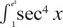dx =

3. The side of a square is increasing at a constant rate of 0.4 cm/sec. In terms of the perimeter, P, what is the rate of change of the area of the square, in cm2/sec?

4. If f is a vector-valued function defined by f(t) = (sin 2t, sin2 t), then f″(t) =

5. The height of a mass hanging from a spring at time t seconds, where t > 0, is given by h(t) = 12 - 4 cos(2t). In the first two seconds, how many times is the velocity of the mass equal to 0?

6.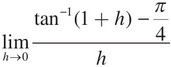7. What is the trapezoidal approximation of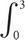ex dx using n = 4 subintervals?

8. Given x2y + x2 = y2 + 1, find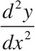at (1, 1).

9. If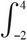f(x) dx = a and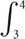f(x) dx = b, then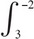f(x) dx =